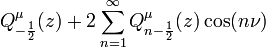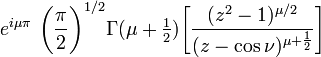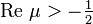User:Tohline/Math/EQ Toroidal08$~ Q_{-\frac{1}{2}}^\mu(z) + 2\sum_{n=1}^\infty Q^\mu_{n-\frac{1}{2}}(z) \cos(n\nu)$$~=$$~ e^{i\mu\pi}~\biggl(\frac{\pi}{2} \biggr)^{1 / 2} \Gamma(\mu + \tfrac{1}{2})\biggl[ \frac{(z^2-1)^{\mu/2}}{(z - \cos\nu)^{\mu + \frac{1}{2}}} \biggr]$ A. Erdélyi (1953):  Volume I, §3.10, p. 166, eq. (3)
 Valid for:$~\mathrm{Re}~\mu > - \tfrac{1}{2}$